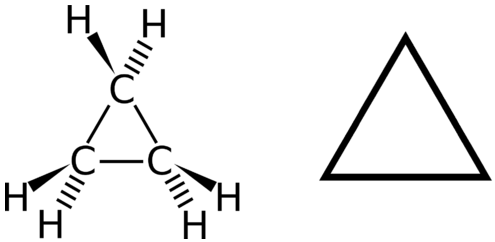# 25.6: Cyclic Hydrocarbons

$$\newcommand{\vecs}{\overset { \rightharpoonup} {\mathbf{#1}} }$$ $$\newcommand{\vecd}{\overset{-\!-\!\rightharpoonup}{\vphantom{a}\smash {#1}}}$$$$\newcommand{\id}{\mathrm{id}}$$ $$\newcommand{\Span}{\mathrm{span}}$$ $$\newcommand{\kernel}{\mathrm{null}\,}$$ $$\newcommand{\range}{\mathrm{range}\,}$$ $$\newcommand{\RealPart}{\mathrm{Re}}$$ $$\newcommand{\ImaginaryPart}{\mathrm{Im}}$$ $$\newcommand{\Argument}{\mathrm{Arg}}$$ $$\newcommand{\norm}{\| #1 \|}$$ $$\newcommand{\inner}{\langle #1, #2 \rangle}$$ $$\newcommand{\Span}{\mathrm{span}}$$ $$\newcommand{\id}{\mathrm{id}}$$ $$\newcommand{\Span}{\mathrm{span}}$$ $$\newcommand{\kernel}{\mathrm{null}\,}$$ $$\newcommand{\range}{\mathrm{range}\,}$$ $$\newcommand{\RealPart}{\mathrm{Re}}$$ $$\newcommand{\ImaginaryPart}{\mathrm{Im}}$$ $$\newcommand{\Argument}{\mathrm{Arg}}$$ $$\newcommand{\norm}{\| #1 \|}$$ $$\newcommand{\inner}{\langle #1, #2 \rangle}$$ $$\newcommand{\Span}{\mathrm{span}}$$

Although cyclohexane can be isolated from petroleum products, a major source of this chemical is the hydrogenation of benzene. Much of the cyclohexane produced is used to manufacture intermediates for the production of nylon. Items such as nylon balloons no doubt had their start in a chemical plant, where hydrogen gas and benzene were reacted at high temperatures to form cyclohexane. This cycloalkane then undergoes nitration to begin the process of forming the long strands of nylon that can be made into balloons, ropes, clothing, and many other useful products.

## Cyclic Hydrocarbons

A cyclic hydrocarbon is a hydrocarbon in which the carbon chain joins to itself in a ring. A cycloalkane is a cyclic hydrocarbon in which all of the carbon-carbon bonds are single bonds. Like other alkanes, cycloalkanes are saturated compounds. Cycloalkanes have the general formula $$\ce{C_{n}H_{2n}}$$. The simplest cycloalkane is cyclopropane, a three-carbon ring.Figure $$\PageIndex{1}$$: Cyclopropane is the simplest cycloalkane. Its highly strained geometry makes it rather unstable and highly reactive.

The structural formulas of cyclic hydrocarbons can be represented in multiple ways, two of which are shown above. Each atom can be shown as in the structure on the left from the figure above. A convenient shorthand is to omit the element symbols and only show the shape, as in the triangle on the right. Carbon atoms are understood to be the vertices of the triangle.

The carbon atoms in cycloalkanes are still $$sp^3$$ hybridized, with an ideal bond angle of $$109.5^\text{o}$$. However, an examination of the cyclopropane structure shows that the triangular structure results in a $$\ce{C-C-C}$$ bond angle of $$60^\text{o}$$. This deviation from the ideal angle is called ring strain and makes cyclopropane a fairly unstable and reactive molecule. Ring strain is decreased for cyclobutane, with a bond angle of $$90^\text{o}$$, but is still significant. Cyclopentane has a bond angle of about $$108^\text{o}$$. This minimal ring strain for cyclopentane makes it a more stable compound.Cyclohexane is a six-carbon cycloalkane, shown below.All three of the depictions of cyclohexane are somewhat misleading, because the molecule is not planar. In order to reduce the ring strain and attain a bond angle of approximately $$109.5^\text{o}$$, the molecule is puckered. The puckering of the ring means that every other carbon atom is above and below the plane. The figure below shows two possibilities for the puckered cyclohexane molecule. Each of the structures is called a conformation. The conformation on the left is called the chair conformation, while the one on the right is called the boat conformation.Figure $$\PageIndex{2}$$: Chair (left) and boat (right) conformations for cyclohexane.

While both conformations reduce the ring strain compared to a planar molecule, the chair is preferred. This is because the chair conformation results in fewer repulsive interactions between the hydrogen atoms. However, interconversion readily occurs between the two conformations.

Larger cycloalkanes also exist, but are less common. Cyclic hydrocarbons may also be unsaturated. A cycloalkene is a cyclic hydrocarbon with at least one carbon-carbon double bond. A cycloalkyne is a cyclic hydrocarbon with at least one carbon-carbon triple bond. Shown below are the simplified structural formulas for cyclohexene and cyclooctyne.## Summary

• A cyclic hydrocarbon is a hydrocarbon in which the carbon chain joins to itself in a ring.
• A cycloalkane is a cyclic hydrocarbon in which all of the carbon-carbon bonds are single bonds. (Like other alkanes, cycloalkanes are saturated compounds.)
• A cycloalkene is a cyclic hydrocarbon with at least one carbon-carbon double bond.
• A cycloalkyne is a cyclic hydrocarbon with at least one carbon-carbon triple bond.
• Names and structures of typical cyclic hydrocarbons are given.

This page titled 25.6: Cyclic Hydrocarbons is shared under a CK-12 license and was authored, remixed, and/or curated by CK-12 Foundation via source content that was edited to the style and standards of the LibreTexts platform; a detailed edit history is available upon request.